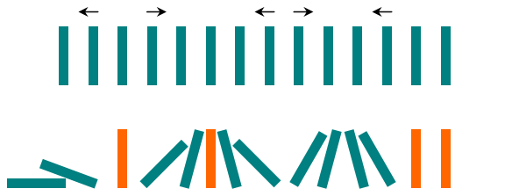838. Push Dominoes
Medium
3.1K
196

There are `n` dominoes in a line, and we place each domino vertically upright. In the beginning, we simultaneously push some of the dominoes either to the left or to the right.

After each second, each domino that is falling to the left pushes the adjacent domino on the left. Similarly, the dominoes falling to the right push their adjacent dominoes standing on the right.

When a vertical domino has dominoes falling on it from both sides, it stays still due to the balance of the forces.

For the purposes of this question, we will consider that a falling domino expends no additional force to a falling or already fallen domino.

You are given a string `dominoes` representing the initial state where:

• `dominoes[i] = 'L'`, if the `ith` domino has been pushed to the left,
• `dominoes[i] = 'R'`, if the `ith` domino has been pushed to the right, and
• `dominoes[i] = '.'`, if the `ith` domino has not been pushed.

Return a string representing the final state.

Example 1:

```Input: dominoes = "RR.L"
Output: "RR.L"
Explanation: The first domino expends no additional force on the second domino.
```

Example 2:```Input: dominoes = ".L.R...LR..L.."
Output: "LL.RR.LLRRLL.."
```

Constraints:

• `n == dominoes.length`
• `1 <= n <= 105`
• `dominoes[i]` is either `'L'`, `'R'`, or `'.'`.
Accepted
111.9K
Submissions
196.4K
Acceptance Rate
57.0%

Seen this question in a real interview before?
1/4
Yes
No

Discussion (0)

Related Topics